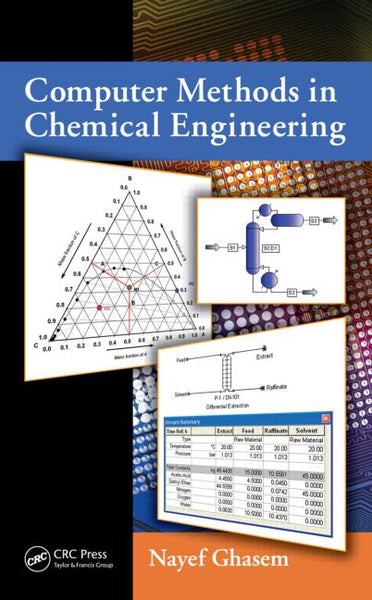# Computer Methods in Chemical Engineering

: In stock

: brown-tech

: 9781439849996

: Book

\$71.96

### Features

• Combines theory, hand calculations, and results obtained from the Hysys/Unisim, PRO/II, Aspen Plus, and SuperPro Designer software packages
• Helps students solve realistic problems and explore alternatives that are inaccessible via hand calculations
• Covers many applications in chemical engineering, including fluid flow in pipes, pumps, and compressors; reactor design; heat exchangers; distillation; absorption; and extraction
• Shows how to solve systems of equations using POLYMATH, E-Z Solve, Simulink, and gPROMS
• Teaches how to generate correlations for experimental data with Excel, SigmaPlot, and SYSTAT
• Explains how to complete a Gantt chart using Microsoft Visio
• Includes problems and references at the end of each chapter

Solutions manual, figure slides, and lecture slides available for qualifying instructors

### Summary

While various software packages have become quite useful for performing unit operations and other kinds of processes in chemical engineering, the fundamental theory and methods of calculation must also be understood in order to effectively test the validity of these packages and verify the results. Computer Methods in Chemical Engineering presents the most commonly used simulation software, along with the theory involved. It covers chemical engineering thermodynamics, fluid mechanics, material and energy balances, mass transfer operations, reactor design, and computer applications in chemical engineering.

Through this book, students learn:

• What chemical engineers do
• The functions and theoretical background of basic chemical engineering unit operations
• How to simulate chemical processes using software packages
• How to size chemical process units manually and with software
• How to fit experimental data
• How to solve linear and nonlinear algebraic equations as well as ordinary differential equations

Along with exercises and references, each chapter contains a theoretical description of process units followed by numerous examples that are solved step by step via hand calculations and computer simulation using Hysys/Unisim, PRO/II, Aspen Plus, and SuperPro Designer. Adhering to the Accreditation Board for Engineering and Technology (ABET) criteria, the book gives students the tools needed to solve real problems involving thermodynamics and fluid-phase equilibria, fluid flow, material and energy balances, heat exchangers, reactor design, distillation, absorption, and liquid–liquid extraction.

• Isbn-13 Digit: 9781439849996